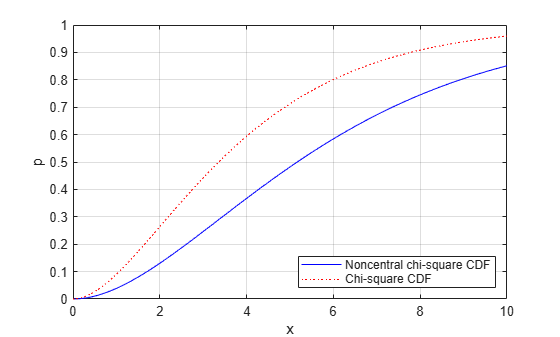ncx2cdf

Noncentral chi-square cumulative distribution function

Syntax

p = ncx2cdf(x,v,delta)
p = ncx2cdf(x,v,delta,'upper')

Description

p = ncx2cdf(x,v,delta) computes the noncentral chi-square cdf at each value in x using the corresponding degrees of freedom in v and positive noncentrality parameters in delta. x, v, and delta can be vectors, matrices, or multidimensional arrays that all have the same size, which is also the size of p. A scalar input for x, v, or delta is expanded to a constant array with the same dimensions as the other inputs.

p = ncx2cdf(x,v,delta,'upper') returns the complement of the noncentral chi-square cdf at each value in x, using an algorithm that more accurately computes the extreme upper tail probabilities.

Some texts refer to this distribution as the generalized Rayleigh, Rayleigh-Rice, or Rice distribution.

The noncentral chi-square cdf is

$F\left(x|\nu ,\delta \right)=\sum _{j=0}^{\infty }\left(\frac{{\left(\frac{1}{2}\delta \right)}^{j}}{j!}{e}^{\frac{-\delta }{2}}\right)\mathrm{Pr}\left[{\chi }_{\nu +2j}^{2}\le x\right]$

Examples

collapse all

Compare the noncentral chi-square cdf with DELTA = 2 to the chi-square cdf with the same number of degrees of freedom (4):

x = (0:0.1:10)';
ncx2 = ncx2cdf(x,4,2);
chi2 = chi2cdf(x,4);

plot(x,ncx2,'b-','LineWidth',2)
hold on
plot(x,chi2,'g--','LineWidth',2)
legend('ncx2','chi2','Location','NW')References

 Johnson, N., and S. Kotz. Distributions in Statistics: Continuous Univariate Distributions-2. Hoboken, NJ: John Wiley & Sons, Inc., 1970, pp. 130–148.